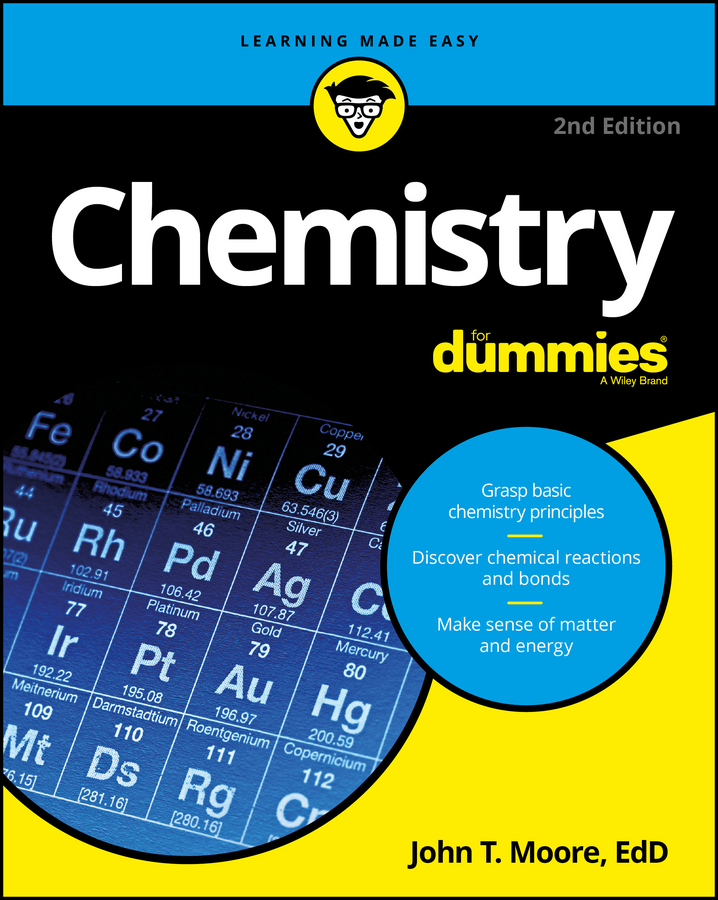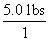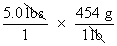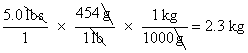##### Chemistry For DummiesScientists are often called on to make measurements of matter, which may include such things as mass (weight), volume, and temperature. A worldwide measurement system has been adopted to ensure that scientists can speak the same language.

## The SI system of scientific measurement

The SI system (from the French Systeme International) is a worldwide measurement system based on the older metric system that most of us learned in school. There are minor differences between the SI and metric systems, but they’re basically interchangeable.

SI is a decimal system with basic units for things like mass, length, and volume, and prefixes that modify the basic units. For example, the prefix kilo- (k) means 1,000. So a kilogram (kg) is 1,000 grams and a kilometer (km) is 1,000 meters.

Two other very useful SI prefixes are centi- (c) and milli- (m), which mean 0.01 and 0.001, respectively. So a milligram (mg) is 0.001 grams — or you can say that there are 1,000 milligrams in a gram.

## SI/English conversions

Many years ago, there was a movement in the U.S. to convert to the metric system. But Americans are still buying their potatoes by the pound and their gasoline by the gallon. Most professional chemists use both the U.S. and SI systems without any trouble. It’s necessary to make conversions when using two systems.

The basic unit of length in the SI system is the meter (m). A meter is a little longer than a yard; there are 1.094 yards in a meter, to be exact. But that’s not a really useful conversion. The most useful SI/English conversion for length is:

2.54 centimeters = 1 inch

The basic unit of mass in the SI system for chemists is the gram (g). And the most useful conversion for mass is:

454 grams = 1 pound

The basic unit for volume in the SI system is the liter (L). The most useful conversion is:

0.946 liter = 1 quart

## The unit conversion method

You’ll find that it’s often unclear how to actually set up chemistry problems to solve them. A scientific calculator will handle the math, but it won’t tell you what you need to multiply or what you need to divide.

That’s why you need to know about the unit conversion method, which is sometimes called the factor label method. It will help you set up chemistry problems and calculate them correctly.

Two basic rules are associated with the unit conversion method:

• Rule 1: Always write the unit and the number associated with the unit. (Rarely in chemistry will you have a number without a unit. The mathematical pi is an exception.)

• Rule 2: Carry out mathematical operations with the units, canceling them until you end up with the unit you want in the final answer. In every step, you must have a correct mathematical statement.

For example, suppose that you have a 5-pound sack of potatoes and you want to know its weight in kilograms. Write down 5 pounds (lbs) as a fraction by placing it over 1.Because you need to cancel the unit lbs in the numerator, you must find a relationship between lbs and something else — and then express that something else with lbs in the denominator. You know the relationship between pounds and grams, so you can use that:Now simply convert from grams to kilograms in the same way: